Statistical and probability models: Represent data effectively

# Unit 3: Frequency polygons and line graphs

Dylan Busa### Unit 3: Frequency polygons and line graphs

By the end of this unit you will be able to:

• Calculate measures of central tendency and percentiles on grouped data.
• Construct a frequency polygon.
• Construct a line graph.

## What you should know

Before you start this unit, make sure you can:

• Calculate measures of central tendency and percentiles. Refer to unit 3 of Subject outcome 4.1 if you need help with this.
• Construct a bar graph. Refer to unit 2 of this Subject outcome if you need help with this.
• Construct a histogram. Refer to unit 2 of this Subject outcome if you need help with this.

## Introduction

In this unit, we will continue to explore some other types of graphs that we can use to visualise data and make it easier to interpret. In particular we will look at how to create frequency polygons and line graphs. See Figures 1 and 2 for examples.

## Measures of central tendency for grouped data

In Subject outcome 4.1 we learnt how to calculate the three main measures of central tendency:

• The mean – the ‘average’ value calculated by dividing the sum of all the values by the number of values.
• The median – the centre-most value of the data set.
• The mode – the value in the data set that occurs most often.

Up until now, we calculated these measures on raw data. However, these values can also be calculated using grouped data.### Example 3.1

The following frequency distribution shows the mass (in kilograms) of a troop of chimpanzees.1. Calculate the approximate mean of the data.
2. Why can we only calculate the approximate mean?
3. What is the median and the median group?
4. What is the mode and the modal group?

Solutions

1. Because we do not have each individual value, we treat each value as though it is at the midpoint of the class. Therefore, we assume that there are seven values of $\scriptsize \displaystyle \frac{{40+45}}{2}=42.5$, $\scriptsize 10$ values of $\scriptsize \displaystyle \frac{{45+50}}{2}=47.5$, and so on. Therefore, the approximate mean of the grouped data is as follows:
\scriptsize \begin {align*}\bar{x}&=\displaystyle \frac{{\left( {42.5\times 7} \right)+\left( {47.5\times 10} \right)+\left( {52.5\times 15} \right)+\left( {57.5\times 12} \right)+\left( {62.5\times 6} \right)}}{{\left( {7+10+15+12+6} \right)}}\\&=\displaystyle \frac{{297.5+475+787.5+690+375}}{{50}}\\&=52.5\end {align*}
2. We can only approximate the mean because we do not have all the exact values and we must assume that each of the values within each class falls at the midpoint of that class.
3. Just as the median is the middle value of ungrouped data, the median of grouped data is the midpoint of the middle class. In this case, the middle class is $\scriptsize 50 \lt m\le 55$ so the median is $\scriptsize 52.5$. The median group is $\scriptsize 50 \lt m\le 55$.
4. Just as the mode is the most frequent value of ungrouped data, the mode of grouped data is the midpoint of the class with the greatest frequency. In this case, the class with the greatest frequency is $\scriptsize 50 \lt m\le 55$ so the mode is $\scriptsize 52.5$. The modal group is $\scriptsize 50 \lt m\le 55$.### Exercise 3.1

The frequency distribution below shows the time taken (in minutes) for various games in a chess tournament to be completed.1. Find the mean of the data.
2. What is the modal group?
3. What is the median?

The full solutions are at the end of the unit.

## Frequency polygons

A frequency polygon (see Figure 1) is a graph created by using straight lines to join the midpoints of each interval, or class in a frequency distribution. The height of each point represents the frequency of the class. The midpoint of a class is calculated by dividing the sum of the upper and lower boundaries by two.

A frequency polygon can also be created from a histogram. You will often see the frequency polygon superimposed over the histogram (see Figure 3).

Because a polygon is a closed shape, the beginning and end of the frequency polygon must touch the x-axis. To do this, we must add a class below and above the classes we have for the data, and must join the midpoints of these classes as well. Because these classes or intervals are empty (frequency is zero), these dots lie on the x-axis.### Example 3.2

The NC(V) mathematics marks, out of $\scriptsize 50$ , for $\scriptsize 35$ learners are given below:

$\scriptsize 46, 40, 12, 10, 47, 23, 26, 8, 29, 34, 37, 17, 40, 50, 18, 23, 33, 23, 24, 15, 35, 23, 19, 22, 28, 35, 27, 42, 29, 26, 46, 33, 27, 19, 28$

1. Complete the table below using the above marks.2. Construct a histogram for these data.
3. If the pass mark is $\scriptsize 21$ out of $\scriptsize 50$, what percentage of learners passed the test?
4. Construct the frequency polygon for these data.
5. Comment on any trends you may see in the graphs.

Solutions

1. .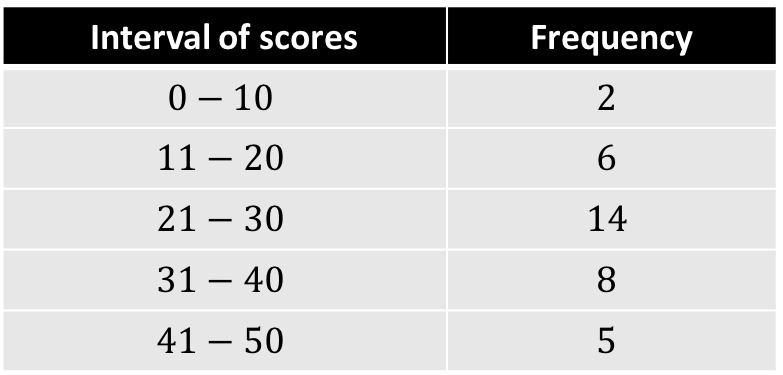2. .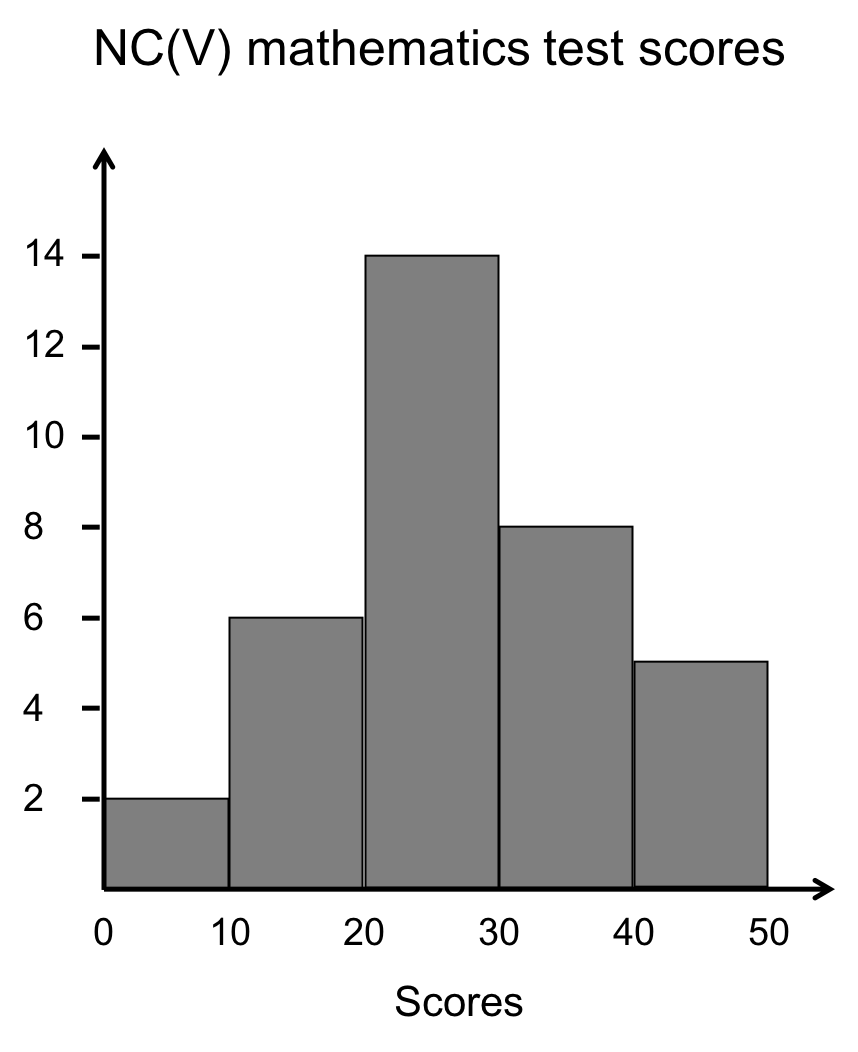3. A total of $\scriptsize 35$ learners wrote the test. A total of $\scriptsize 14+8+5=27$ learners scored $\scriptsize 21$ out of $\scriptsize 50$ or more. Therefore, the percentage of learners who passed is $\scriptsize \displaystyle \frac{{27}}{{35}}\times 100=77.14\%$.
4. To construct the frequency polygon, we need to join dots at the midpoints of each of the classes or intervals. However, we also need to close the polygon by adding dots at the midpoints of the intervals below and above the data intervals i.e. the intervals of $\scriptsize -10-0$ and $\scriptsize 51-60$. The frequency polygon is drawn as follows: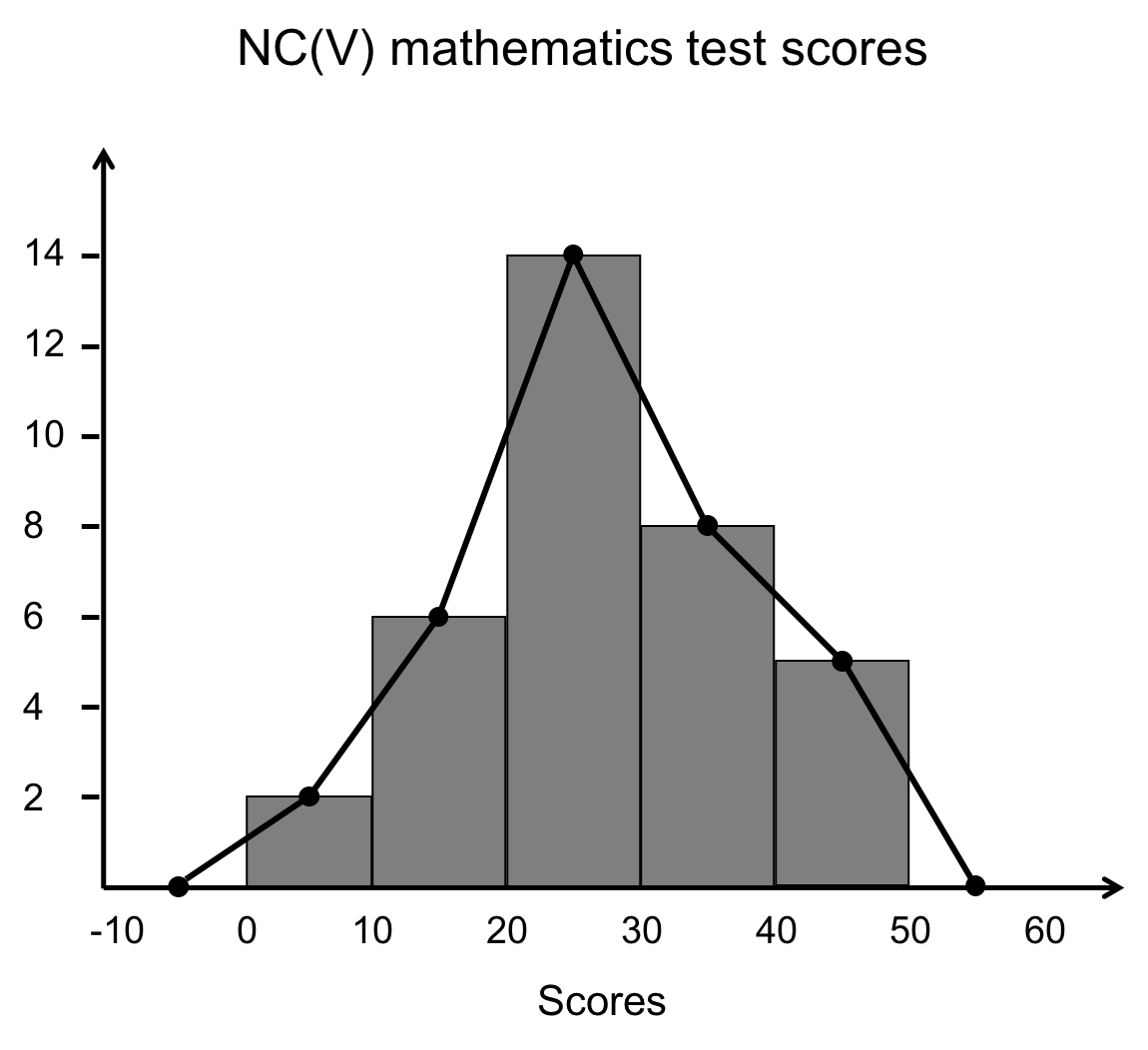5. The data follows a normal distribution but there is a slight skew to the right with more students scoring above $\scriptsize 30$ marks than would otherwise be expected.### Exercise 3.2

The following frequency distribution is for the heights (in $\scriptsize \text{cm}$) of a group of $\scriptsize 90$ students.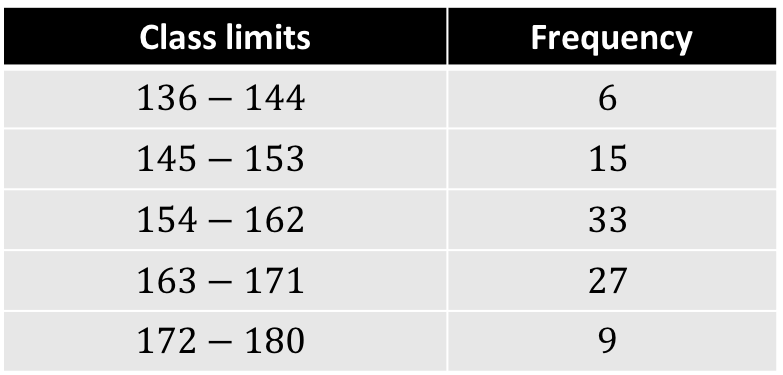1. Create a frequency polygon for this data.
2. Calculate the mean of this data.
3. What is the median of this data?
4. What is the mode of this data?

The full solutions are at the end of the unit.

## Line graphs

Another common way to display numerical (discrete and continuous quantitative) data is by using a line graph. You may be familiar with line graphs already. In many ways they are similar to frequency polygons but are not based on grouped data.

They are most often used to show changes or trends over time but what they show is the relationship between an independent variable (like time) and a dependent variable that depends on the independent variable.

In a line graph, each data value is represented by a point on the graph. The points are then connected by straight lines. The independent variable is listed along the horizontal, or x-axis, and the quantity or value of the data is listed along the vertical, or y-axis.

### Note

Watch the short video called “Line Graphs: Lesson (Basic Probability and Statistics Concepts)” for a basic introduction to line graphs.

Line Graphs: Lesson (Basic Probability and Statistics Concepts) (Duration: 04.44)### Activity 3.1: Draw a line graph

Time required: 10 minutes

What you need:

• a pen or pencil
• a piece of paper
• a ruler

What to do:

The table below shows the height of a certain tree (in metres) for each year between 2003 and 2009.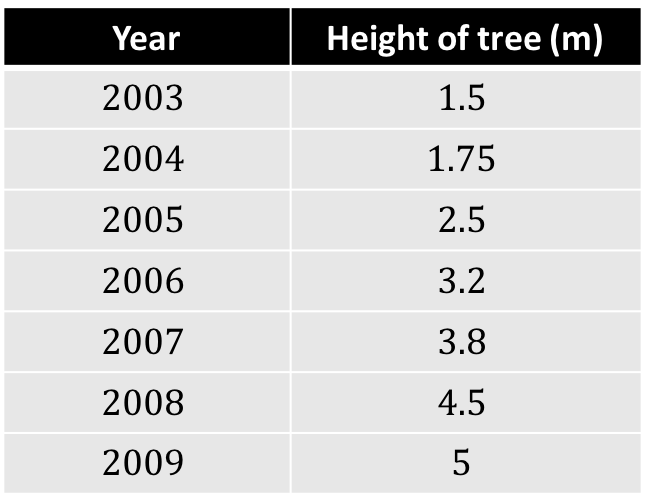1. Draw a set of axes. You only need to draw the positive portions of the x- and y-axes. Make each axis at least $\scriptsize 20\ \text{cm}$ long.
3. Now determine the units on the y-axis. Look at the smallest and largest values in the table and determine the best scale for this axis. Mark and label these points on your y-axis.
4. Evenly divide your x-axis among the years in the table and label these marks.
5. Now plot each of your data points as accurately as possible and draw straight lines between each of these points.
6. State any conclusions you can draw from your graph.
7. How does your line graph differ from a frequency polygon?

What did you find?

After working through points 1 to 5 this is what your line graph should look like.1. The rate of growth of the tree has been steady since 2004. Between 2003 and 2004, its rate of growth was lower, possibly due to a lack of water or nutrients.
2. This line graph differs from a frequency polygon in two important ways. Firstly, there is no need for the graph to start and end on the x-axis. Secondly, the points on the graph represent actual data values rather than a frequency of values within a particular interval.

### Note

If you have an internet connection, spend time playing with the wonderful line graph simulation called Understand and Create Line Graphs: Line Graphs. Here you can practise creating a line graph and answer some questions about it.### Exercise 3.3

The table below shows South Africa’s real Gross Domestic Product (GDP) growth rate (in percentage terms) between 2007 and 2017.1. Draw a line graph of these data.
2. What was the general trend in South Africa’s economic performance over this period?
3. In which year was the highest rate of growth?
4. What was the mean growth rate over this period?
5. Draw a horizontal line on your graph representing the mean growth rate.
6. In which year(s) was the growth rate below the mean?

The full solutions are at the end of the unit.

## Summary

In this unit you have learnt the following:

• How to calculate the mean, median and mode of grouped data.
• How to create a frequency polygon.
• How to create a line graph.

# Unit 3: Assessment

#### Suggested time to complete: 30 minutes

1. The frequency distribution below shows the number of passengers that travel in Alfred’s minibus taxi per week.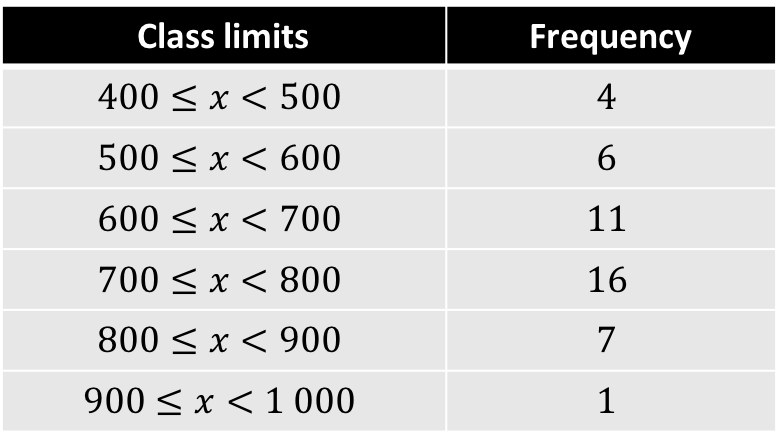1. Create a frequency polygon of this data.
2. What is the modal interval?
3. How many weeks does this data cover?
4. Calculate an estimate for the total number of passengers to travel in Alfred’s taxi over the entire period?
5. Give an estimate of the mean number of passengers per week.
6. If it is estimated that every passenger travelled an average distance of $\scriptsize 5\ \text{km}$, how much money would Alfred have taken in an average week if he charged $\scriptsize \text{R}3.50$ per kilometre?
2. The table gives the average fuel price per litre (in Rands) in South Africa for unleaded 95 octane petrol (ULP 95) between 2007 and 2017.1. Create a line graph to represent this data.
2. What overall trend do you see in the data?

The full solutions are at the end of the unit.

# Unit 3: Solutions

### Exercise 3.1

1. We will need to calculate the mean of grouped data. To do this we assume that each value within a class or interval is at the midpoint of the interval.
\scriptsize \begin {align*}\bar{x}&=\displaystyle \frac{{\left( {5\times 40} \right)+\left( {12\times 50} \right)+\left( {15\times 60} \right)+\left( {28\times 70} \right)+\left( {18\times 80} \right)+\left( {14\times 90} \right)+\left( {7\times 100} \right)}}{{99}}\\&=71.31\ \min \end {align*}
2. The modal group is the group, class or interval with the highest count or frequency. This is $\scriptsize 65 \lt t\le 75$.
3. The median is calculated as the midpoint of the middle group, class or interval. The median class is $\scriptsize 65 \lt t\le 75$. Therefore, the median is $\scriptsize 70$.

Back to Exercise 3.1

### Exercise 3.2

1. .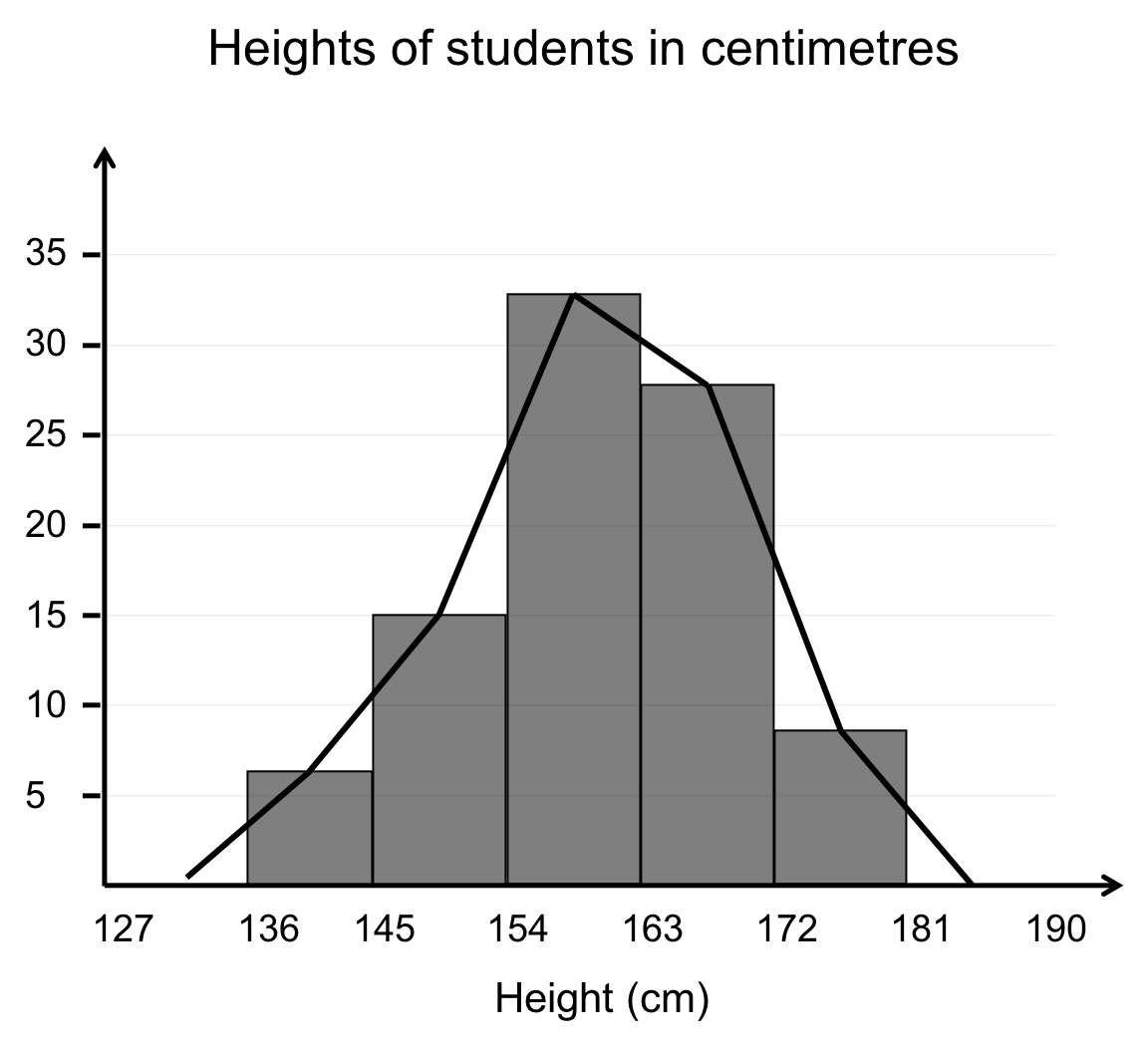or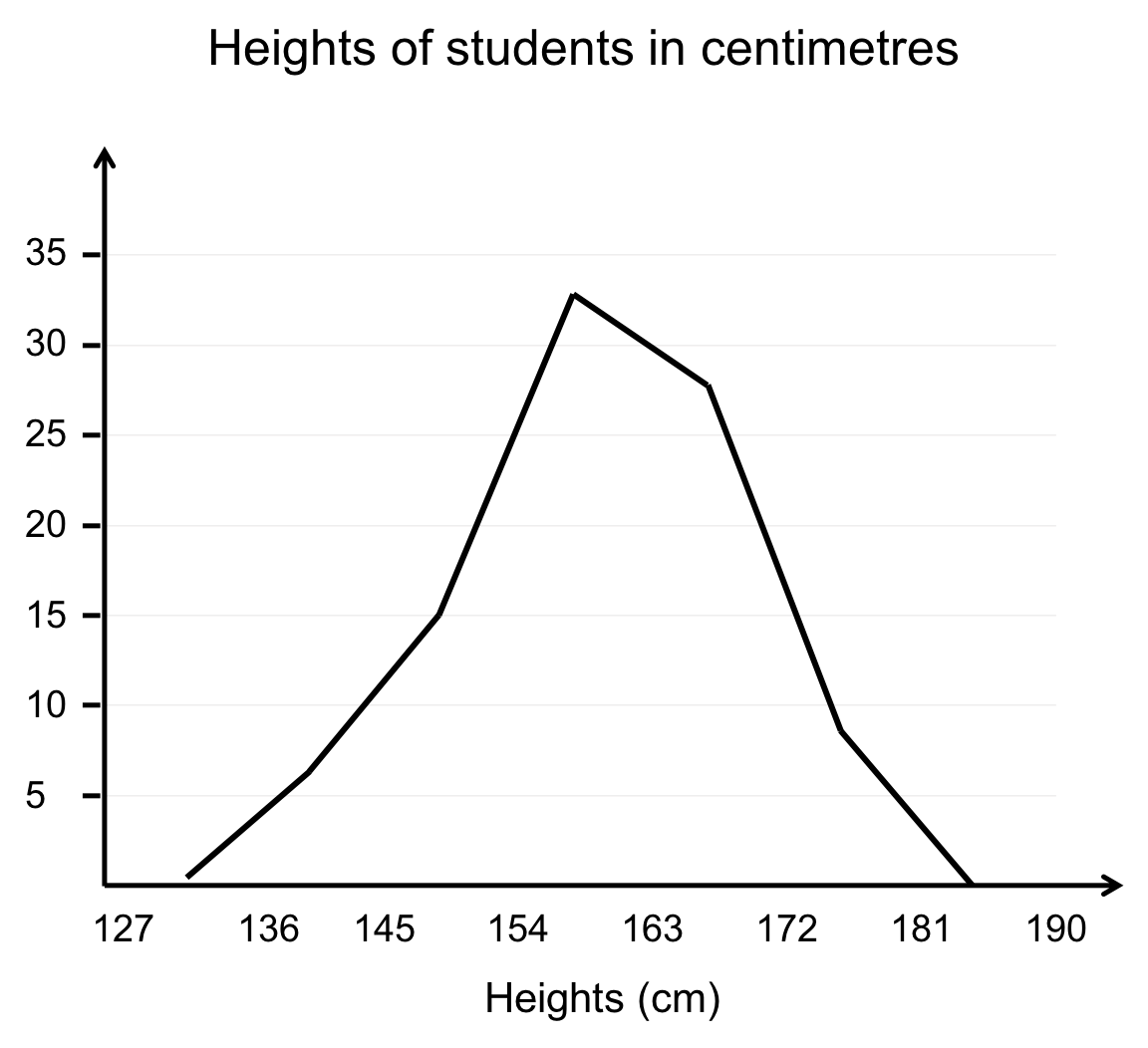2. We do not have the original raw data so we will need to calculate the mean of the grouped data. To do this we assume that each value within a class or interval is at the midpoint of the interval.
\scriptsize \begin {align*}\bar{x}&=\displaystyle \frac{{\left( {6\times 140} \right)+\left( {15\times 149} \right)+\left( {33\times 158} \right)+\left( {27\times 167} \right)+\left( {9\times 176} \right)}}{{90}}\\&=159.8\ \text{cm}\end {align*}
3. The median is the midpoint of the median class or media interval. The median class is $\scriptsize 154-162$. Therefore, the median is $\scriptsize 158$.
4. The mode is the midpoint of the modal class or modal interval. The modal interval is $\scriptsize 154-162$. Therefore, the mode is $\scriptsize 158$.

Back to Exercise 3.2

### Exercise 3.3

1. In this set of data there was a negative value. This means that the graph extends below the x-axis for a period.2. The general trend was a decline in GDP growth over the period, especially between 2012 and 2016.
3. The highest growth rate was in 2007.
4. Mean growth rate:
\scriptsize \begin {align*}\bar{x}&=\displaystyle \frac{{5.4+3.2-1.5+3.0+3.3+2.2+2.5+1.8+1.3+0.6+1.3}}{{11}}\\&=2.1\end {align*}
5. .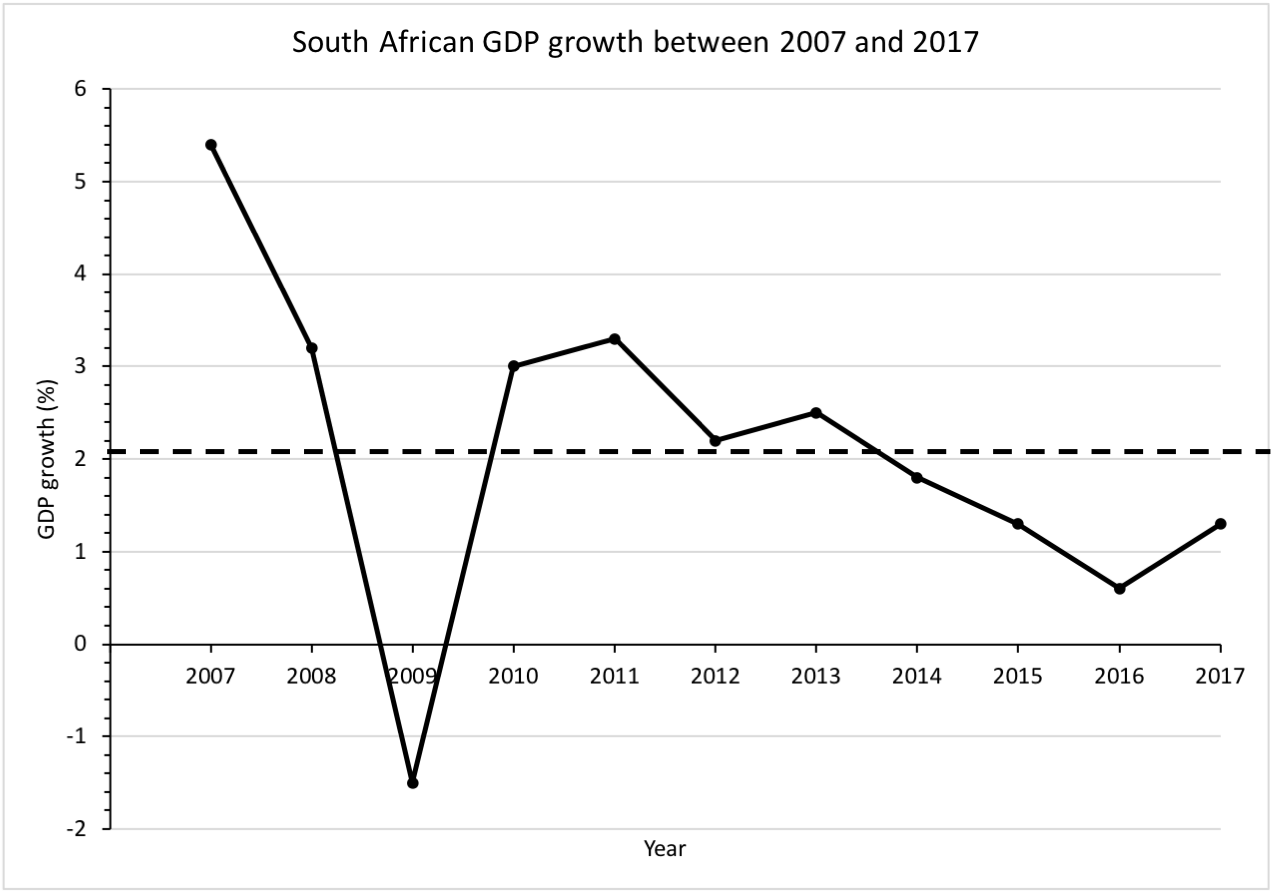6. The growth rate was below the mean in 2009, and from 2014 to 2017.

Back to Exercise 3.3

### Unit 3: Assessment

1. .
1. .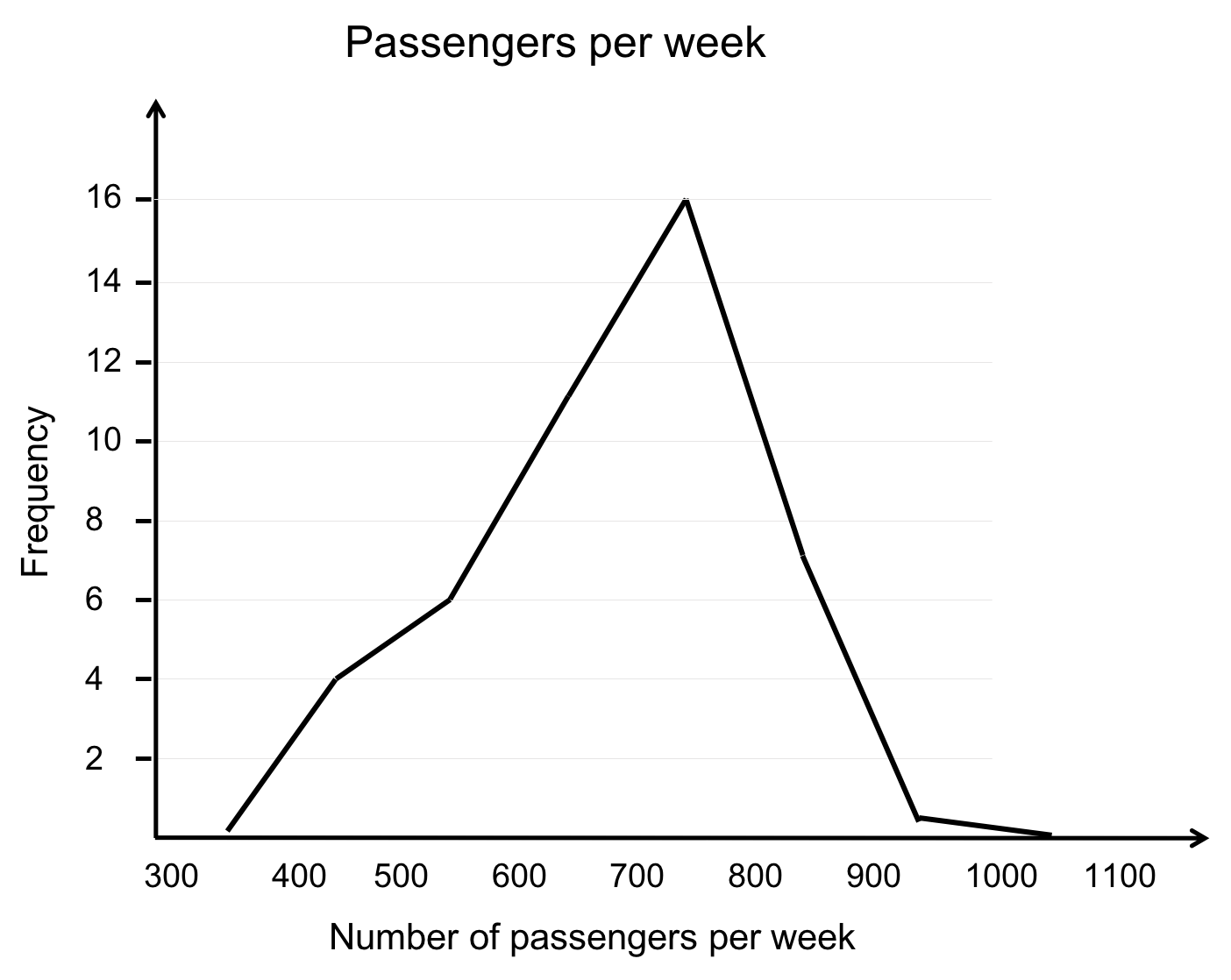2. The modal interval is $\scriptsize 700-799$.
3. The data covers $\scriptsize 4+6+11+16+7+1=45$ weeks.
4. Estimated total number of passengers:
$\scriptsize \left( {4\times 450} \right)+\left( {6\times 550} \right)+\left( {11\times 650} \right)+\left( {16\times 750} \right)+\left( {7\times 850} \right)+\left( {1\times 950} \right)=31\ 150$
5. $\scriptsize \bar{x}=\displaystyle \frac{{31150}}{{45}}=692$ passengers per week
6. $\scriptsize 692\ \text{passengers}\times 5\ \text{km }\times \text{R}3.50/\text{km}=\text{R}12\ 110/\text{week}$.
2. .
1. .2. The overall trend is an increase in the price of ULP 95 over the period.

Back to Unit 3: Assessment